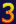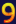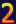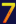"The direct use of force is such a poor solution to any problem. It is generally employed only by small children and large nations."
- David Friedman

Friday, May 1, 2009 | Permalink

In the comments to Shader programming tips #4 Java Cool Dude mentioned that for fullscreen passes you can pass an interpolated direction vector and use the linearized depth to compute the world position. Basically with view_z computed using the math in #4 you compute:

float3 world_pos = cam_pos + In.dir * view_z;

This amounts to only two scalar operations and one float3 to carry out.

What about regular non-fullscreen passes? Turns out you can do that as well using a nice DX10 feature. The problem is that we need to interpolate the direction vector in screen space, rather than doing perspective correction. For screen aligned primitives it's the same thing, so it works out in this case, but for "normal" mesh data it's a completely different story. In DX10, and also in GLSL, there's now a noperspective keyword you can add to your interpolator, which changes the interpolation mode to eliminate the perspective correction, thus giving you an interpolation that's linear in screen space instead.

How do we compute the direction vector? Just take the position you're writing out from the vertex shader and push it to the far plane. Depending on if you're using a reversed projection matrix or not you either want Z=0 or Z=1, which can be done with using float4(Out.Position.xy, 0, Out.Position.w) or Out.Position.xyww respectively in homogenous coordinates. Transform this vector with the inverse view_proj matrix to get the world position of the far plane equivalent of the point in world space. Now subtract cam_pos from this and that's the direction vector. Instead of subtracting the cam_pos in the vertex shader you can just bake that into the same matrix and get it for free. The resulting vertex shader snippet for this is something like this:

float4 dir = mul(view_proj_inv, Out.position.xyww);
Out.dir = dir.xyz / dir.w;

Finally, note that view_z as computed in #4 goes from 0 at the camera to far_plane at the far clipping plane. For this computation we need it to be 1.0 at the far clipping plane, which can be done by simply multiplying ZParams with far_plane. Alternatively you can divide Out.dir with far_plane in the vertex shader.

Name

Comment

Enter the code below